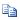# Python标准库11 多进程探索 [multiprocessing包]

XAMPP案例 87浏览

### 进程池“三个进程的进程池”```import multiprocessing as mul

def f(x):
return x**2

pool = mul.Pool(5)
rel  = pool.map(f,[1,2,3,4,5,6,7,8,9,10])
print(rel)```apply_async(func,args)  从进程池中取出一个进程执行func，args为func的参数。它将返回一个AsyncResult的对象，你可以对该对象调用get()方法以获得结果。

close()  进程池不再创建新的进程

join()   wait进程池中的全部进程。必须对Pool先调用close()方法才能join。```www.sina.com.cn
www.163.com
www.iciba.com
www.cnblogs.com
www.qq.com
www.douban.com```### 共享资源``````# modified from official documentation
````import multiprocessing````
``````def f(n, a):
``````    n.value   = 3.14
````    a      = 5````
``````num   = multiprocessing.Value('d', 0.0)
````arr   = multiprocessing.Array('i', range(10))````
``````p = multiprocessing.Process(target=f, args=(num, arr))
``````p.start()
````p.join()````
``````print num.value
````print arr[:]````

Manager

Manager对象类似于服务器与客户之间的通信 (server-client)，与我们在Internet上的活动很类似。我们用一个进程作为服务器，建立Manager来真正存放资源。其它的进程可以通过参数传递或者根据地址来访问Manager，建立连接后，操作服务器上的资源。在防火墙允许的情况下，我们完全可以将Manager运用于多计算机，从而模仿了一个真实的网络情境。下面的例子中，我们对Manager的使用类似于shared memory，但可以共享更丰富的对象类型。

````import multiprocessing````
``````def f(x, arr, l):
``````    x.value = 3.14
``````    arr = 5
````    l.append('Hello')````
``````server = multiprocessing.Manager()
``````x    = server.Value('d', 0.0)
``````arr  = server.Array('i', range(10))
````l    = server.list()````
``````proc = multiprocessing.Process(target=f, args=(x, arr, l))
``````proc.start()
````proc.join()````
``````print(x.value)
``````print(arr)
````print(l)````

Manager利用list()方法提供了表的共享方式。实际上你可以利用dict()来共享词典，Lock()来共享threading.Lock(注意，我们共享的是threading.Lock，而不是进程的mutiprocessing.Lock。后者本身已经实现了进程共享)等。这样Manager就允许我们共享更多样的对象。